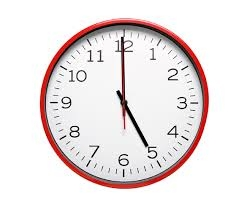# Kilometer 58281

Write a fraction, which part:
1) kilometer is 31 m
2) minutes is 42 seconds
3) the day is 10 o'clock

a =  31/1000
b =  7/10
c =  5/12

### Step-by-step explanation:Did you find an error or inaccuracy? Feel free to write us. Thank you!

Tips to related online calculators
Need help to calculate sum, simplify or multiply fractions? Try our fraction calculator.
Do you want to convert length units?
Do you want to convert time units like minutes to seconds?